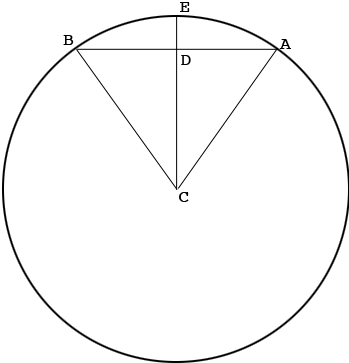SEARCH HOMEMath Central Quandaries & QueriesQuestion from Doug: Specifically, what is the subject distance for the Earth orbiting for 27 days. Assume the orbit of the Earth to be a circle have a 93 million mile radius. Assume the angle of arc to be (27/365) x 360 degrees. Thank you.Hi Doug,

The subject of your email was "Distance between a chord and its arc on a circle" so that is what I'll try to answer. Let me know if this is not what you want.

In my diagram the arc $AB$ is path of the Earth over 7 days and the radius of the circle is 93 million miles. The "Distance between a chord and its arc on a circle" is the length of the line segment $ED.$As you pointed out the measure of the angle $BCA$ is $\large \frac{7}{365} \normalsize \times 360^{0} = 6.90^{0}.$ Hence the measure of the angle DCA$is half of this. The angle$ADC$is a right angle and thus $\cos(DCA) = \frac{|DC|}{|CA|}$ and hence $|DC| = 93,000,000 \times cos\left( \frac{6.90}{2}\right) = 93,000,000 \times 0.998 = 92,831,254 \mbox{ miles.}$ Thus$|ED| = 93,000,000 - 92,831,254 = 168,746\$ miles.

You can't put much faith in this number since the path of the Earth is not a circle and the radius of the "circle" is only approximately 93 million miles.

Penny* Registered trade mark of Imperial Oil Limited. Used under license.Math Central is supported by the University of Regina and the Imperial Oil Foundation.